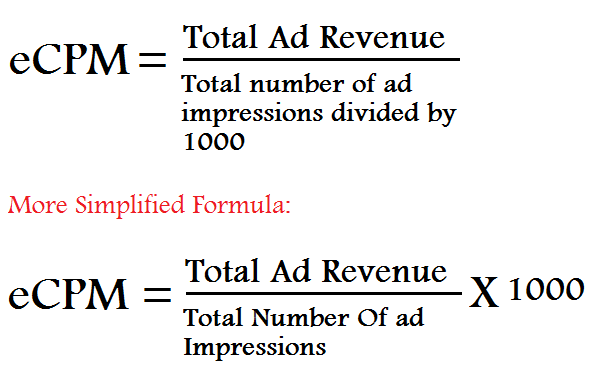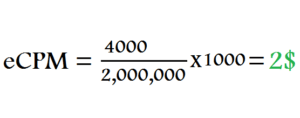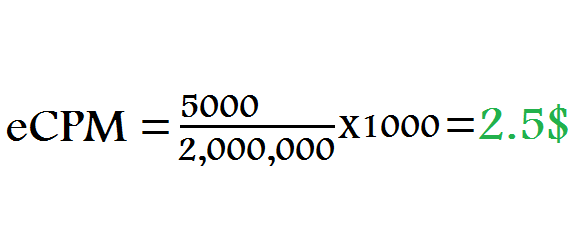# What Is eCPM and How It Is Calculated ?The acronym eCPM stands for “Effective Cost Per Mille“. It is the outcome of a calculation of the ad revenue/earnings generated by an ad campaign, divided by the number of ad impressions of that campaign expressed in units of 1,000.

* Here “Mille” stands for 1000 impressions. Mille is a Latin word which means 1000. If you want to understand what CPM is, then you can follow the below link:

Lets make it more simple through its mathematical formula:To Enlarge The Images Please Click On Them

Don’t worry if you still find it difficult, i will make it more simple to understand with examples, just keep reading the article.

The calculation of eCPM differs for different pricing models (CPM, CPC, CPA), so, i will discuss them one by one.

Page Contents

### Calculating eCPM For A CPM Campaign:

Calculating eCPM for a CPM campaign is more than easy as it is identical to the CPM rate offered by the advertiser or ad network.

For Example: Suppose an advertiser offers 2\$ as CPM rate for his campaign, which means he will pay you 2\$ for per 1000 ad impressions. So, the eCPM would also be 2\$.

Lets calculate it mathematically:

To calculate eCPM we need “Total Ad Revenue” and “Total Number Of ad impressions“. So, first we will find these two things.

Total Ad Revenue = Number of blocks of 1000 impressions X CPM rate.

Calculating Total Number Of Blocks of 1000 impressions:

Number Of Blocks Of 1000 impressions = Total number of impressions delivered / 1000.

So, lets calculate it:

Suppose you have delivered 2 million ad impressions in one day. And CPM rate is 2\$.

So, Number Of Blocks Of 1000 Impressions = 2,000,000/1000 = 2000

Total Ad Revenue = 2000 X 2 = 4000\$

Total Number Of Ad Impressions = 2,000,000

So, our eCPM would be:You can clearly see, the eCPM is same as CPM rate, so, there’s no need to calculate eCPM for CPM campaigns.

### Calculating eCPM For a CPC Campaign:

Suppose, you delivered 2 million ad impressions and generated 5000 clicks and the CPC rate is 1\$. Then, we calculate our eCPM like this:

Total Ad Revenue = Total number of clicks X CPC Rate

= 5000 X 1 = 5000

Total Number Of Ad Impressions = 2,000,000

So, Our eCPM would be:### Calculating eCPM For A CPA Campaign:

Calculating eCPM for a CPA based campaign is also easy. Suppose, you have delivered 2 million impressions and generated 100 leads/sales/conversions and CPA rate is 40\$.

Total Ad Revenue = Total number of conversions X CPA rate

= 100 X 40 = 4000\$

Total Number Of Impressions = 2,000,000

So, our eCPM would be:Now, i think you have understood what eCPM is, but do you know eCPM is not the right term for publishers 😉 .

Almost all ad networks show eCPM for publishers but then why i am saying eCPM is not the right term for publishers. As you know eCPM stands for effective cost per mille but there’s no cost involve for publishers and therefore it is a term for advertisers.

The correct term for publishers is eRPM as they generate revenue.

### What Is eRPM ? And How It Is Different From eCPM ?

The acronym eRPM stands for “Effective Revenue Per Mille“. Both eCPM and eRPM means the same and have a same calculation formula. The only difference is about how we think of it. Advertisers look for the cost involved in advertising and therefore, they think in terms of eCPM whereas publishers look for revenue and therefore, they think in terms of eRPM.

Because both of the terms mean the same and give similar values on calculation, so, ad networks started using a common term eCPM and therefore, it is most commonly used by all – advertisers, publishers and ad networks.

I hope you have enjoyed this article, i tried to be as simple as possible, so, everybody can understand these terms. And, don’t forget to rate this article so that i can understand whether you understood the terms or not. It will help me in making this article better.

Thanks all and happy earnings 🙂

Recommended Articles:

1.Aini